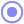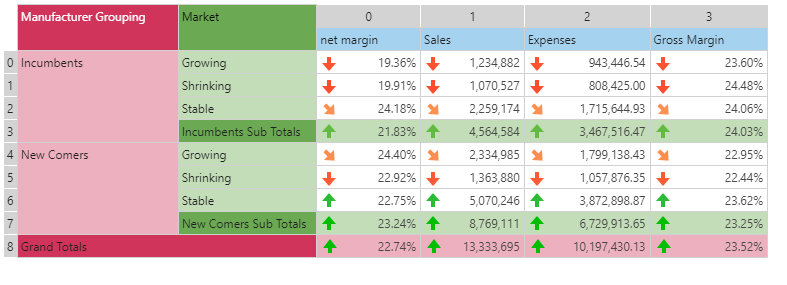#If (PQL)

Evaluates a logical expression, returning the one result if true, or another if false.

• Returned Output: Variant
• Library: PQL \ Common \ Logical
• Version: 2018.00.000
• Compatibility:
• Can be combined with and other PQL function throughout the application.
• It CANNOT be used with MDX or VBA functions. But it can be used on MDX-based content in other parts of the application.

#### Syntax

If( <Criteria> , <TRUE> , <FALSE> )

* Click on the function's arguments above for more details on the input values.

• The criteria expression is a logical statement that evaluates to true or false
• Outcomes can be alphanumeric ("variant") values or embedded functions that resolve to values.
• Click here to review the functional operators covering arithmetic, comparison, and logical operations.
• Logical operators used for composite logical and comparative expressions: && = 'AND';  || = 'OR'
##### Different Function types
• This function is like the Excel "If" function.
• Also see the Case and IfNull functions.

#### Examples

This example checks if 2 is greater than 1. If it is, it returns 3 otherwise 4. In this case, it returns true - so 3.

If( 2>1, 3, 4 )

This example uses the dynamic grid below.

If( data(0,0)>= average(dataSetColumn(0)), "We're doing well", "We're doing badly" )

The logical expression check if data point (0,0) is greater than or equal to the average of the values in column 0. So it compares 19.36% to the average of net margin across all cells. Since the statement turns out to be false, the function resolves to "We're doing badly".